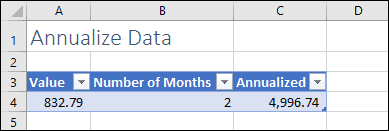# A formula or easy way to annualize data based on month

Annualizing data in Excel is easy if you understand basic Excel formulas and how annualization is calculated.

If Excel formulas are unfamiliar to you, you could benefit greatly from our completely free Basic Skills E-book, which teaches the basics of Excel formulas.

## An Excel formula to annualize data

To annualize data from a single month, the formula will be:

=[Value for 1 month] * 12

This works because there are 12 months in a year.

If you had 2 months of data, the formula would be:

=[Value for 2 months] * 6

This works because there are 6 periods of 2 months in a year.

You can apply this to any number of months by simply dividing 12 by the number of months. In other words, this formula:

=[Value for X months] * (12 / [Number of months])

Many more Excel formulas and functions are covered in our Essential Skills and Expert Skills Books and E-books.